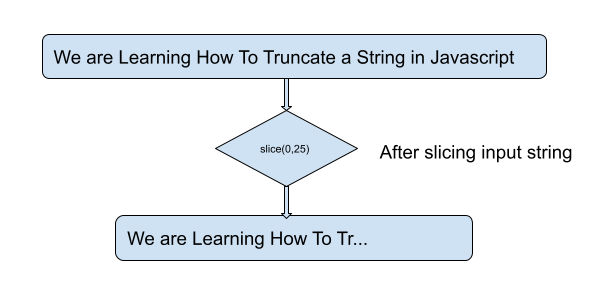# Truncating a String in JavaScript

In the given problem statement we are asked to truncate a string with the help of javascript functionalities. We can solve this problem with the help of Javascript’s built−in functions slice() and substr().

## What do you mean by truncating a string ?

Let's understand the meaning of truncating a string.

Truncating a string means cutting the length of a string by removing character from the beginning, end or middle part of the input string. The aim of truncating a string is to make a certain space or to make it more readable by truncating unnecessary information from the input source.

For example, If we have a long string for an article, we might want to remove it to a shorter length so that it gets fit easily on our article without wrapping to multiple lines or by taking too much space.## Logic for The Above Problem

Let's understand the logic to create the above problem statement:

We have to truncate the given input string to the certain length given in the function and show it on the browser. For doing this we will use some built−in functions of Javascript.

In Javascript we can truncate a string using the slice() method and substr() method. The slice() function returns a new string that contains a portion of the original string, and pass starting index and ending at the specified index. While substring also works similar to the slice() method but this method specifies the end index, we specify the length of the substring we want to extract.

### Algorithm − Using slice() method

Step 1 − Define a string variable named str which contains the long string.

Step 2 − Using the above string and pass this string to the slice method. Starting index 0 to ending index 20. This step will truncate the given string from 0th index to 20 index.

Step 3 − After truncating string in the above step, console the output.

### Example

// define a string to truncate
const str = "I am writing a string to truncate in Javascript";
const truncatedStr = str.slice(0, 20) + "...";
//After truncating the given string
console.log(truncatedStr);


### Output

I am writing a strin...


### Algorithm − Using substr() method

Step 1 − Define a variable of string data type and name it str which contains the long string.

Step 2 − Use the above string and pass this string to the substr() function which is Javascript's built−in function. Starting index 0 to ending index 25. This step will truncate the given string from 0th index to 20 index.

Step 3 − After truncating string in the above step, console the output.

### Example

// define long string to truncate
const str = "I am using substr() method to truncate in Javascript";
const truncatedStr = str.substr(0, 25) + "...";
//After truncating the given string
console.log(truncatedStr);


### Output

I am using substr() metho...


### Algorithm

Step 1 − Define a string variable named str which contains the long string.

Step 2 − Use the above string and pass this string to the replace() function which is Javascript's built−in function. It is using regular expressions to truncate till the index 20.

Step 3 − After truncating string in the above step, console the output.

### Example

//define string to truncate
const str = "This is a long string that needs to be truncated";
const truncatedStr = str.replace(/^(.{20}[^\s]*).*/, "\$1...");
//after truncating the given string
console.log(truncatedStr);


### Output

This is a long string...


## Complexity

The time complexity for the above defined methods slice(), substr() and replace is O(n). Here n is the size of the input original string. The reason for this complexity is that these methods iterate over the characters in the string and create a new substring by making a copy of the selected characters.

While the regular expression method has a time complexity of O(k), in this k is the number of characters that match the regular expression pattern. In the third code the regular expression matches the first 20 characters of the string.

## Conclusion

In this code we have implemented different kinds of algorithms using built−in functions in javascript. We can note down here that for practical purposes, the performance differences between these methods are likely to be negligible for most strings of reasonable length. The choice of method to be used can be dependent on factors such as readability and maintainability of the code.

Updated on: 23-Aug-2023

2K+ Views